# Electric Current Current Electricity Current Electricity is the

• Slides: 17Electric CurrentCurrent Electricity • Current Electricity is the flow of an electric charge through a conductor.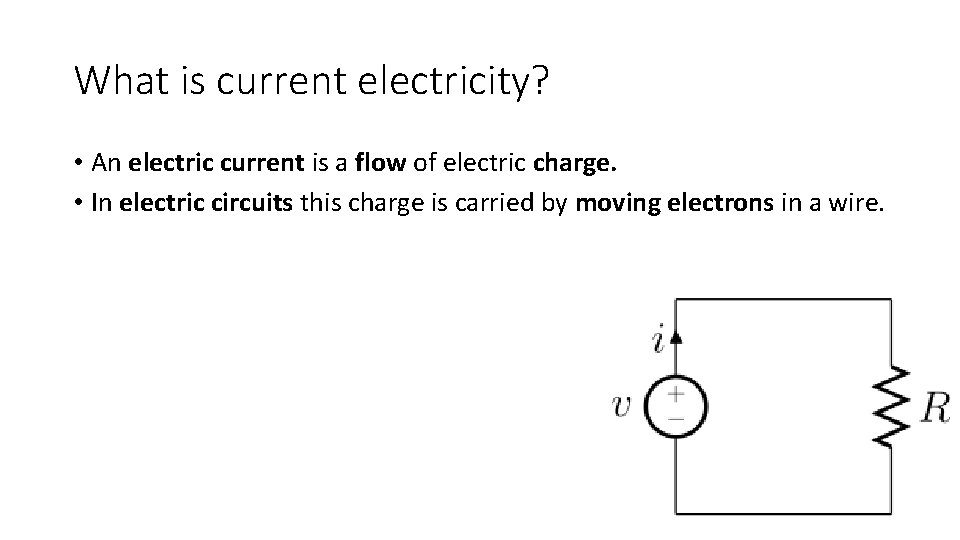What is current electricity? • An electric current is a flow of electric charge. • In electric circuits this charge is carried by moving electrons in a wire.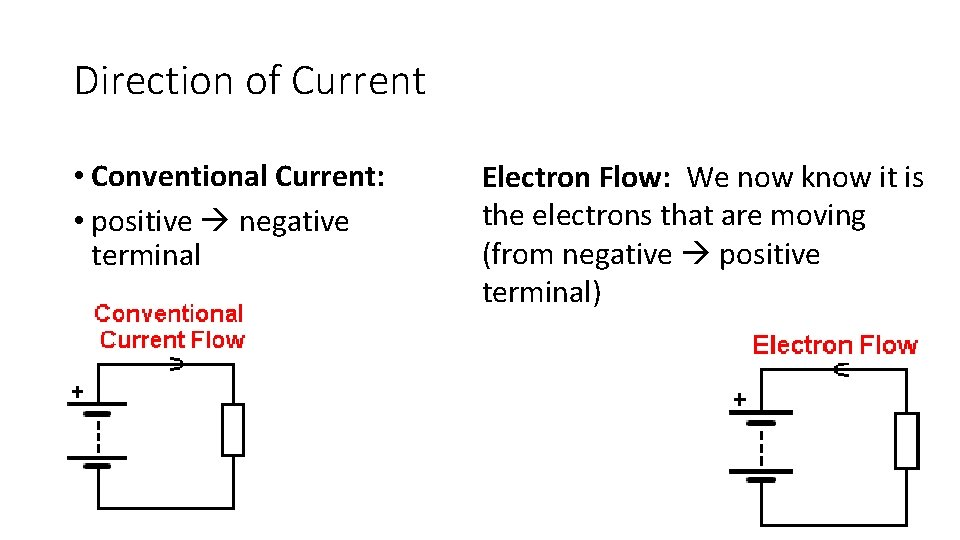Direction of Current • Conventional Current: • positive negative terminal Electron Flow: We now know it is the electrons that are moving (from negative positive terminal)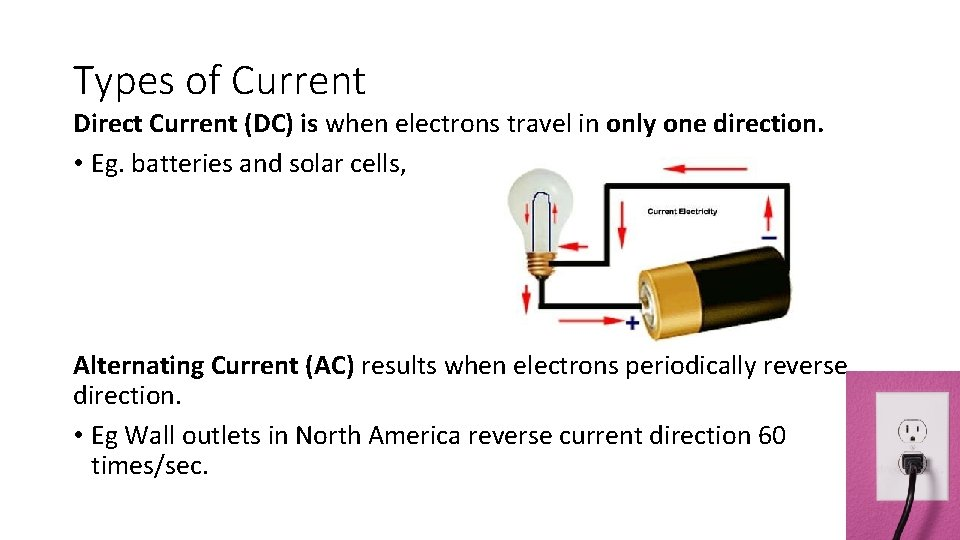Types of Current Direct Current (DC) is when electrons travel in only one direction. • Eg. batteries and solar cells, Alternating Current (AC) results when electrons periodically reverse direction. • Eg Wall outlets in North America reverse current direction 60 times/sec.Measuring Electric Current (I) • Electric current is the amount of charge passing through a point in a circuit in a given time. • Measured in amperes (A) • 1 Amp is the typical amount of current flowing through a lightbulb. • Ammeter measures current at one point in a circuit.Calculating Current • Current (I) is expressed by the equation: • Q = quantity of charge flowing by a point in a time period of t. • A current of 1 ampere = 1 Coulomb /second. • 1 Coulomb is equal to the charge contained in 6. 25 x 10 18 electrons • 1 ampere represents the current of one coulomb of charge per second.Calculating how many electrons go past a point in a set amount of time Q=IxtPractice An ammeter reads 10 A. How many electrons flow through the circuit in 1 min? 6. 25 x 10 18 electrons/C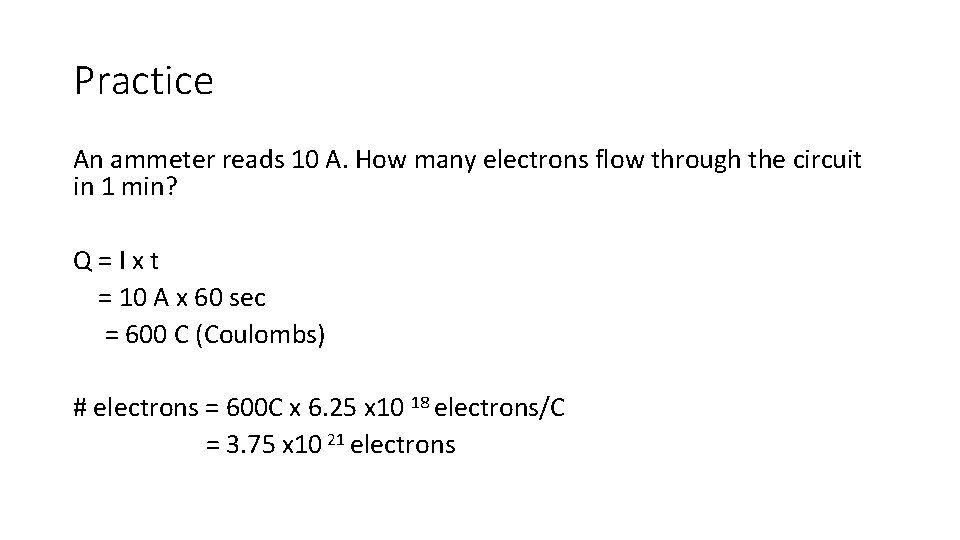Practice An ammeter reads 10 A. How many electrons flow through the circuit in 1 min? Q = I x t = 10 A x 60 sec = 600 C (Coulombs) # electrons = 600 C x 6. 25 x 10 18 electrons/C = 3. 75 x 10 21 electrons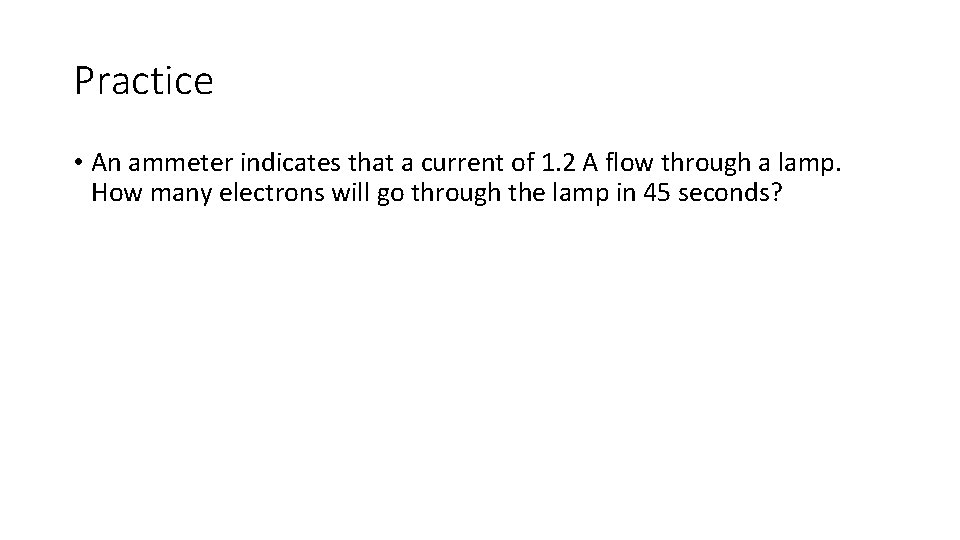Practice • An ammeter indicates that a current of 1. 2 A flow through a lamp. How many electrons will go through the lamp in 45 seconds?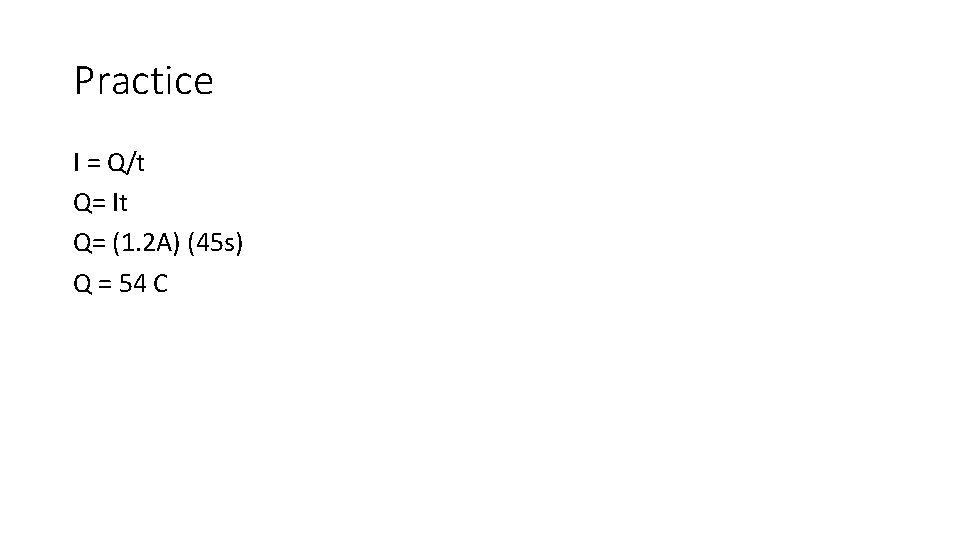Practice I = Q/t Q= It Q= (1. 2 A) (45 s) Q = 54 C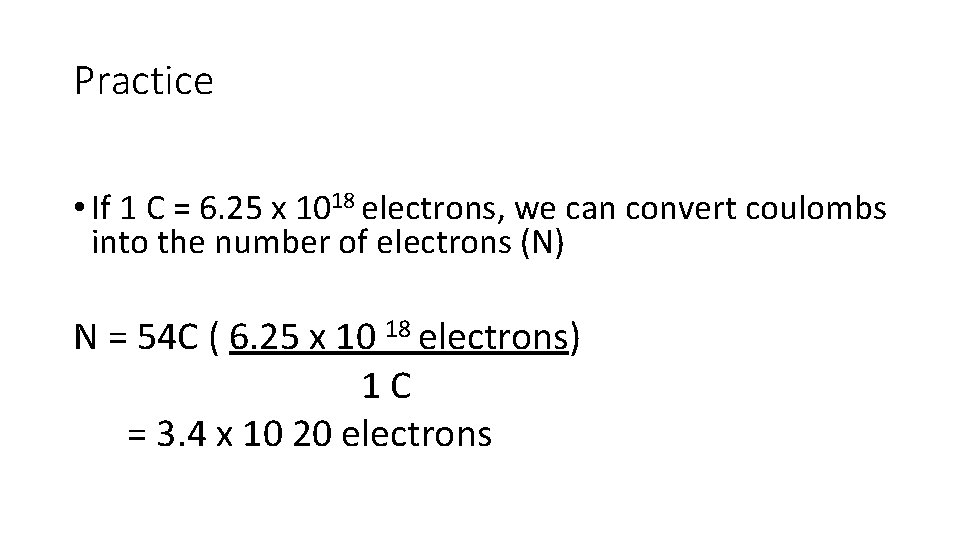Practice • If 1 C = 6. 25 x 1018 electrons, we can convert coulombs into the number of electrons (N) N = 54 C ( 6. 25 x 10 18 electrons) 1 C = 3. 4 x 10 20 electrons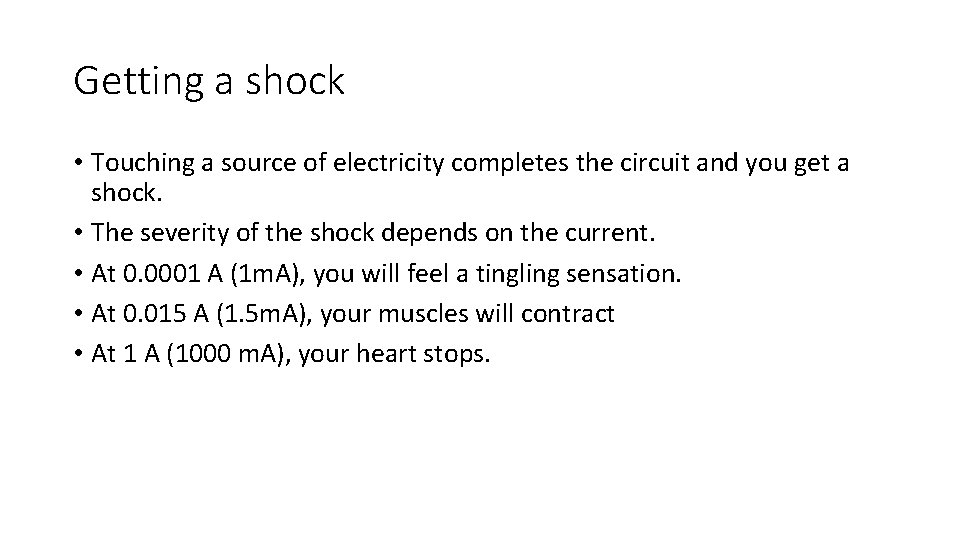Getting a shock • Touching a source of electricity completes the circuit and you get a shock. • The severity of the shock depends on the current. • At 0. 0001 A (1 m. A), you will feel a tingling sensation. • At 0. 015 A (1. 5 m. A), your muscles will contract • At 1 A (1000 m. A), your heart stops.Circuits in Series • only one path for electrons to flow. • Electricity must flow through all devices • If you add electric loads, each on get less electric pressure (light bulbs will dim as more added) • We use series circuits in: • Computers, radios, TV’s, rockets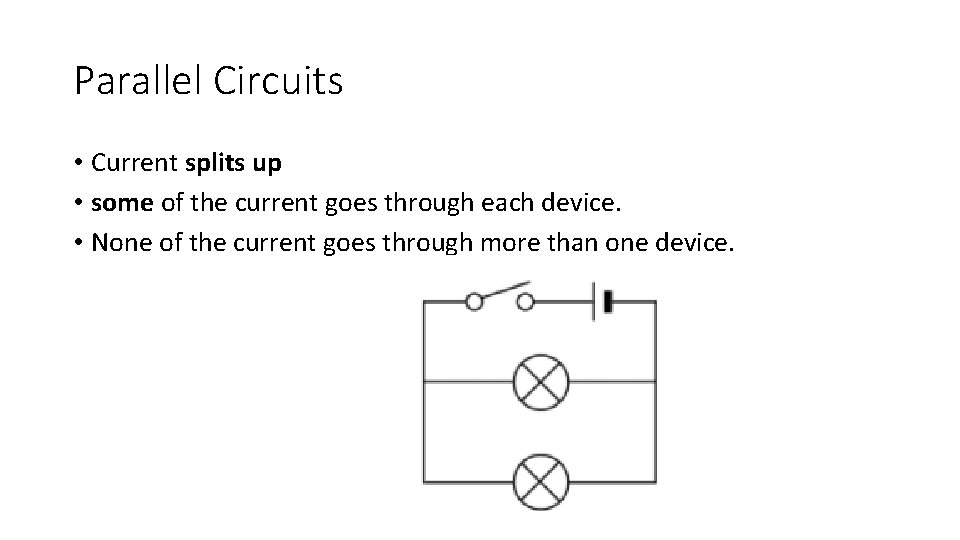Parallel Circuits • Current splits up • some of the current goes through each device. • None of the current goes through more than one device.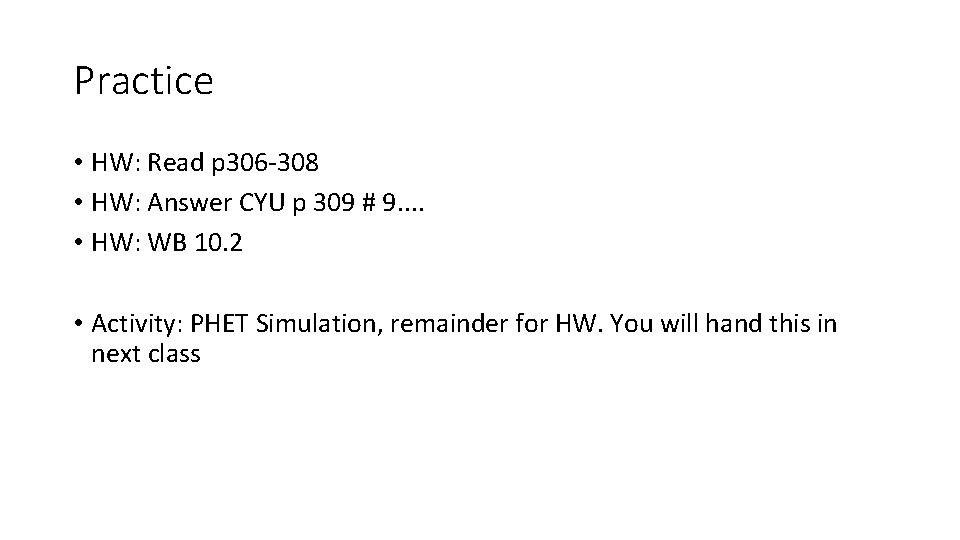Practice • HW: Read p 306 -308 • HW: Answer CYU p 309 # 9. . • HW: WB 10. 2 • Activity: PHET Simulation, remainder for HW. You will hand this in next class